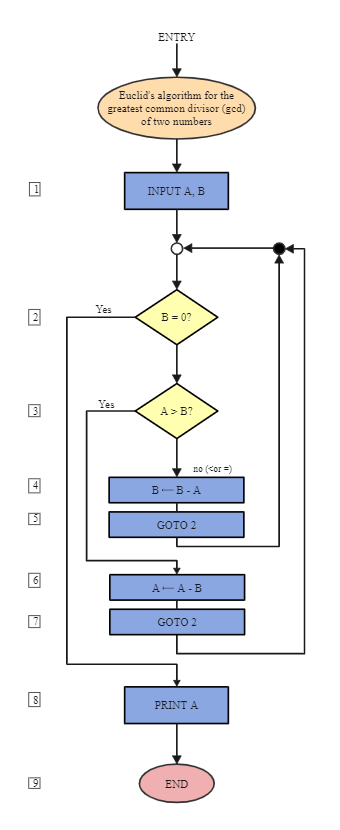Template Community / Euclid Common Divisor Algorithm Flowchart

# Euclid Common Divisor Algorithm FlowchartCharlotte
Published on 2021-07-16
Edit OnlineAs illustrated in the below image, the Euclidean algorithm or Euclid's algorithm is an efficient method for computing the greatest common divisor of two integers, the most significant number that divides them both without a remainder. To better understand the below algorithm, let us take an example: 21 is the greatest common divisor of 252 and 105, and the same number 21 is also the GCD of 105 and 252-105 = 147. Since the replacement reduces the larger of the two numbers, repeating this process gives successively smaller numbers until the two numbers become equal. When this occurs, they are the greatest common divisor of the original two numbers. By reversing the steps, the GCD can be expressed as a linear combination of the two original numbers, the sum of the two numbers.
Tag
algorithm flowchart
Report
0
587PostRecommended Templates